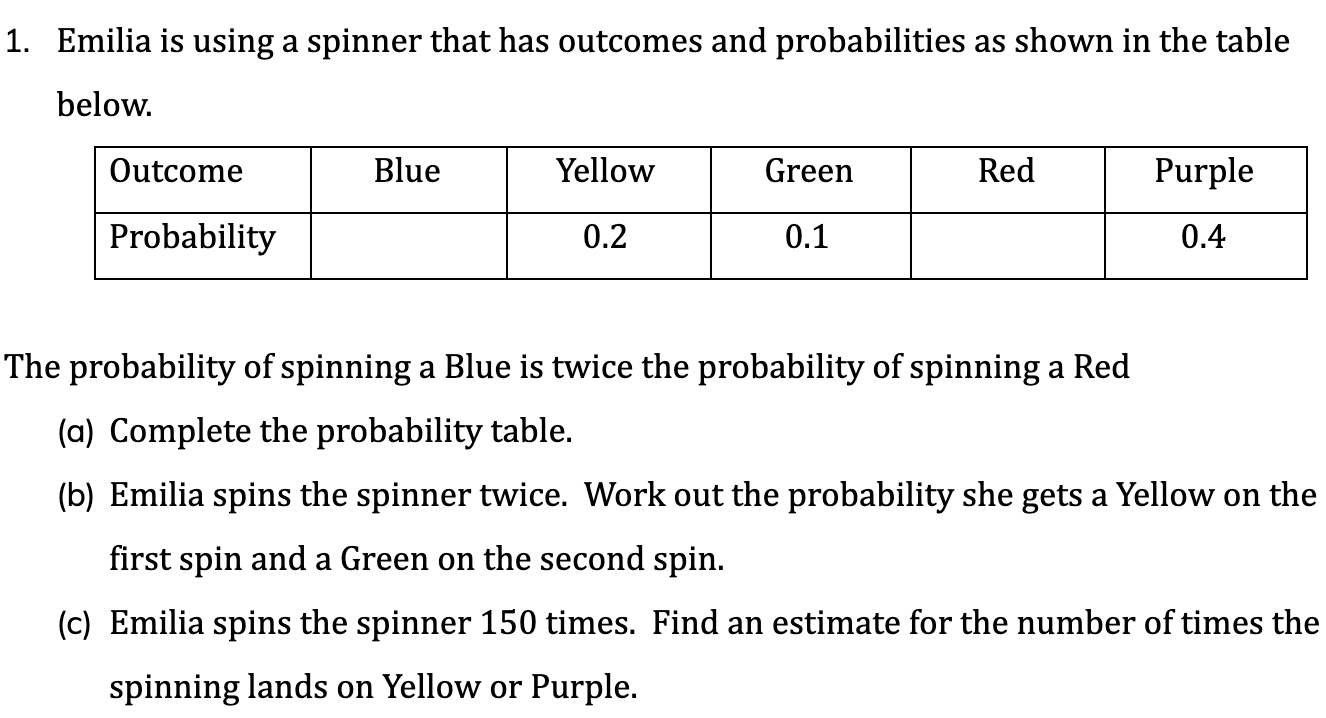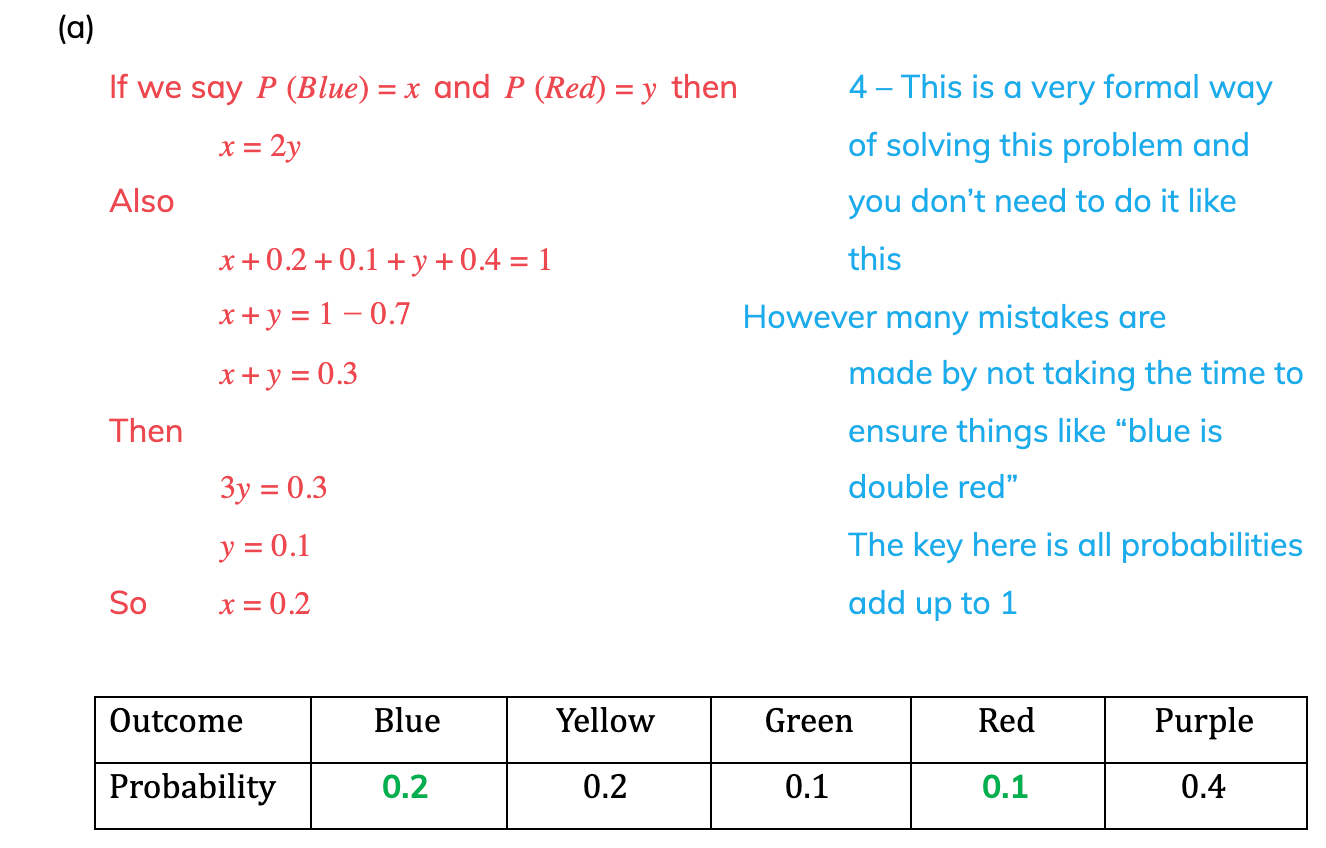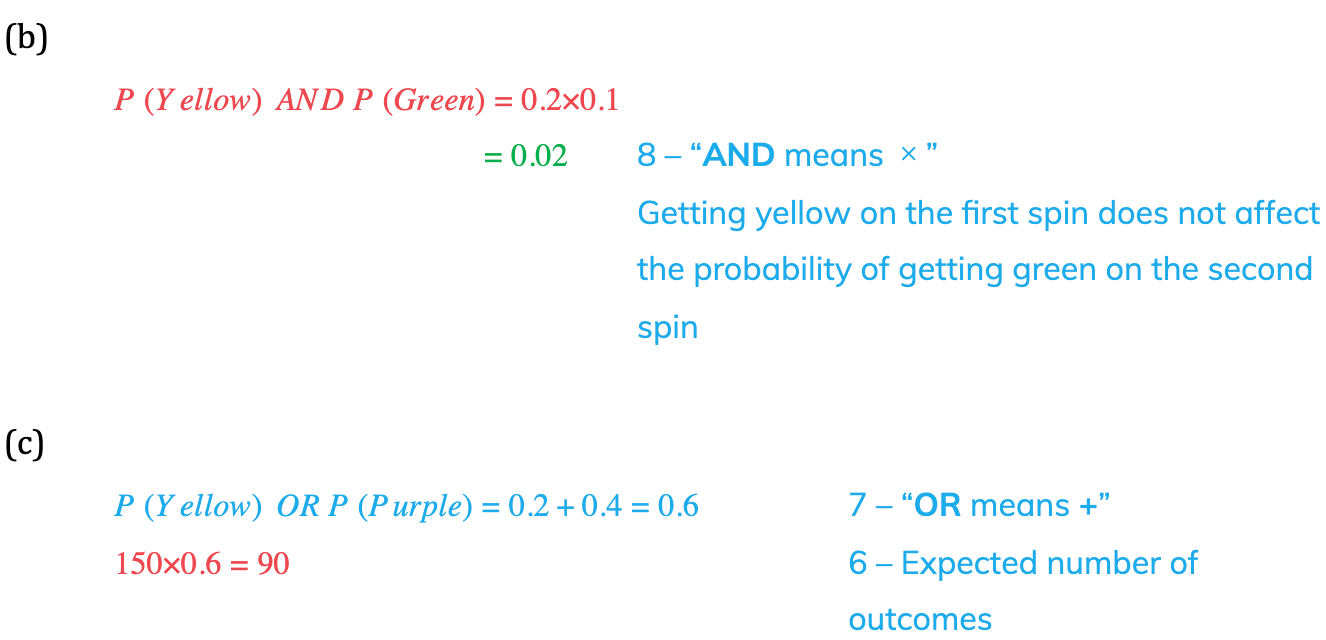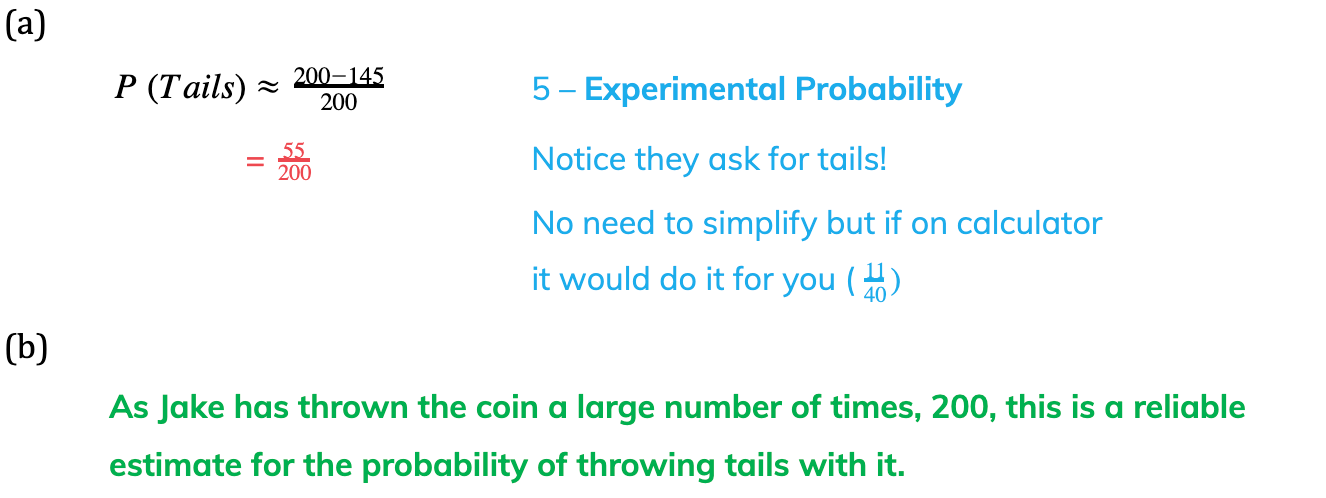# Edexcel IGCSE Maths 复习笔记 6.4.1 Basic Probability

Edexcel IGCSE Maths 复习笔记 6.4.1 Basic Probability

#### What do we mean by basic probability?

• There are both simple concepts and very hard topics in probability
• Probability is used in many areas and industries, eg. insurance costs and the rate of interest on loans

#### What do I need to know?

• Be aware of how we write and use probability notation:

1. Event

• When we have a trial or experiment there may be several outcomes
• We use capital letters to denote an event – this is something that might happen in our trial
• For example, A could be the event of getting an even number when rolling a dice
• Note that it is more common to see reference to “outcomes” rather than “events” but they broadly mean the same thing.

2. “A-dash”

• If A is an event, then A’ (spoken: “A-dash”) is the event “not A”
• Notice we’re not necessarily interested in what has happened, just that A hasn’t

3. Probability of ...

• P(A) means the probability that event A happens (eg. rolling a six on a dice)
• P(A’) means the probability that event A does not happen (eg. getting any other number when rolling a dice)
• The letter n is often used to talk about the number of times A happens (or might happen) if the trial is repeated several times

4. Total probability

• All the different events for a trial have a total probability of 1 (certainty)
• This should make sense in that something will happen from a trial (eg. when rolling a (normal) dice it is certain you will get a number between 1 and 6)

5. Experimental probability

• Also known as theoretical probability
• This is where the probability of an event can be determined by considering all the possible outcomes (eg. on a dice we would say the probability of getting each individual number is 1/6) without performing any trials
• However sometimes we don’t know this (maybe because we know we have a biased dice but don’t know how it is biased) so we can only talk about probabilities once we have done some trials:• The more trials that are done, the more the experimental probability will reflect the true probability of the event

6. Number of outcomes

• If we want to estimate the number of times an event will happen out of a total of n trails we calculate:

No. of times A happens=n ×P(A)

• You will sometimes see/hear this being called the “expected number of times A happens”

7. Mutually exclusive (OR means +)

• Mutually exclusive events cannot happen at the same time, rolling a 2 on a dice and rolling a 4 on a dice
• This leads to the result that8. Independent events (AND means )

• This is the first situation where we might combine different events from different trials
• For example:A is the event “Rolling a 3” and B is the event “Flipping heads”These events are independent because one does not affect (the probability of) the other

So to find the probability of both events happening we multiply their individual probabilities together:• Note that this is NOT the opposite of mutually exclusive – because both often crop up together it is easy to think they must be linked

#### Exam Tip

It is unusual in probability questions that you will be asked to simplify fractions – so don’t, in case you mess it up! You could use your calculator to do it automatically but this topic can appear on all papers.In probability questions, it is usually easiest to use whatever number format the question does. Probabilities can be fractions, decimals or percentages (nothing else!). If no format is indicated in the question then fractions are normally best.

#### Worked Example#### Worked Example#### Worked Example#### Worked Example